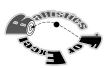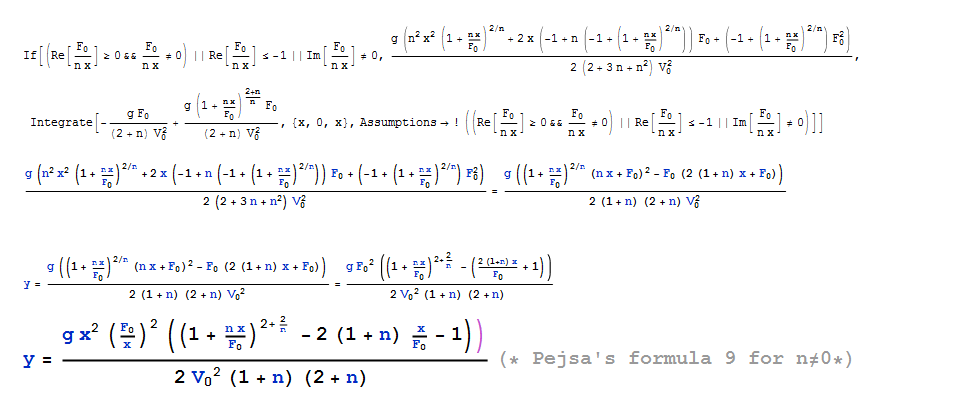# Forum

Home Ballistics for Excel Visit the BfX forumBfX is based on the book of Arthur Pejsa. Pejsa deploys the Siacci method for approximating bullet trajectories. Here one takes a flat-fire approximation for trajectories. That makes the math easier, but limits the elevations to a maximum of 15 degrees. Beyond this angle the approximation becomes too inaccurate for some use-cases. Another characteristic of the Siacci method is the parametrization of the drag function by (a few) splines allowing closed form mathematical trajectory calculations. For a discussion on ballistics see the link page and Wikipedia's page on exterior ballistics in English or Dutch. The algorithms that are deployed are derived by myself, following Pejsa's approach. As a consequence some of the typos are not affecting BfX, the formulas have metric constants, some approximations are skipped, the range of applicability extends below the speed of sound and BfX supports many drag functions. The math was done partly with Mathematica, a computer program with which one can manipulate equations.